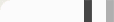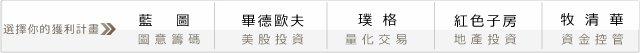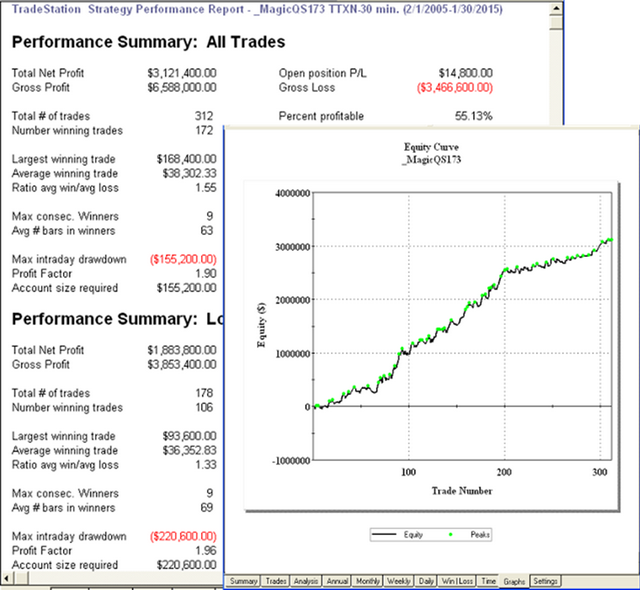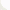## 開發商品的交易系統 - 基礎篇  勁道指數{系統參數與變數}
input:ExitType(1);
vars: IsBalanceDay(False),MP(0),PF(0),PL(0);

inputs: Len1(5),Len2(5),HB(0),LB(0),HighBar(5),LowBar(5);
vars:Force(0),Vol(0),ForceIdx1(0),ForceIdx2(0),Avg1(0),Avg2(0);

MP = MarketPosition ;

if DAYofMonth(Date) > 14 and DAYofMonth(Date) < 22 and DAYofWeek(Date)= 3 then isBalanceDay = True else isBalanceDay =False ;

{ 計算勁道指數 }
Vol = iff(DataCompression > 1 ,Volume,TickS) ;
Force = Vol * (Close-Close) ;
ForceIdx1 = Xaverage(Force,Len1) ;
ForceIdx2 = Xaverage(Force,Len2) ;

{ 作兩次平方根計算只是方便指標圖觀看與參數測試}
ForceIdx1 = sign(ForceIdx1)*SquareRoot(SquareRoot(Absvalue(ForceIdx1))) ;
ForceIdx2 = sign(ForceIdx2)*SquareRoot(SquareRoot(Absvalue(ForceIdx2))) ;

{均線用來協助確認趨勢的方向}
Avg1 = Average(Close,Len1) ;
Avg2 = Average(Close,Len2) ;

1.我使用的進場邏輯有些類似逆勢想法 ，或者說是在趨勢中的反彈與回檔中進場
2.多空不對稱參數與參考界線不同

{多單進場 - 收盤價低於均線且勁道指數高於 HB時進場}
if MP <> 1 and Close < Avg1 and ForceIdx1 > HB then Buy next bar at Highest(High,HighBar) stop ;

{空單進場 - 收盤價高於均線且勁道指數低於 LB時進場}
if MP <> -1 and Close > Avg2 and ForceIdx2 < LB then Sell next bar at Lowest(Low,LowBar) stop ;

if ExitType = 1 then SetStopLoss(PL * BigPointValue) ;

if ExitType = 2 then Begin
SetStopLoss(PL * BigPointValue) ;
setProfitTarget(PF * BigPointValue) ;
end;

if ExitType = 3 then Begin
if MP > 0 and BarsSinceEntry = NBarL then ExitLong next bar at Market ;
if MP < 0 and BarsSinceEntry = NBarS then ExitShort next bar at Market ;
end;

if ExitType = 4 then Begin
SetStopLoss(PL * BigPointValue) ;
setProfitTarget(PF * BigPointValue) ;
if MP > 0 and BarsSinceEntry = NBarL then {Sell } ExitLong next bar at Market ;
if MP < 0 and BarsSinceEntry = NBarS then {Buy} ExitShort next bar at Market ;
end;

if IsBalanceDay or date = 1150224 then setExitonClose ;

#### 台指期 60 min K 多空留倉 交易週期 2005/2/1~ 2015/1/31 交易成本 1200#### 台指期 30 min K 多空留倉 交易週期 2005/2/1~ 2015/1/31 交易成本 1200#### 0 意見: